# Weight Conversion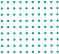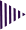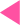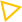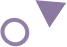=
Best Weight conversion calculator

# Online weight conversion calculator

For converting between different weight units, the weight converter tool is the best option. There is also a real-time weight conversion tool to help you convert between different units, such as pounds to kilograms, kilograms to pounds, and ounces to stones, etc. This article will also explain what weight is, whether it is a force, and even briefly touch on body weight, health issues, and how to lose weight (and gain weight) if you are interested.
An object's weight is determined by the force of gravity pulling it down. Based on the mass of the object and the acceleration due to gravity, which is 9.8 m/s2 on Earth. Weight is calculated by the formula F = m * 9.8 m/s2, where F is the object's weight in Newtons (N) and m is its mass in kilograms.

### How do you calculate weight conversion?

An object's weight is determined by the force of gravity pulling it down. Based on the mass of the object and the acceleration due to gravity, which is 9.8 m/s2 on Earth. Weight is calculated by the formula F = m * 9.8 m/s2, where F is the object's weight in Newtons (N) and m is its mass in kilograms.

### How many gram are in a kilogram?

You need to divide the kilogram figure by 1000 to find the number of grams in a kilogram since a kilogram contains 1000 grams. There are 1 kilograms in 1000 grams.

### Weight Conversion Units Definitions

• Kilogram (kg) converter definition:-

In the international system of Units (SI), the metric system, kilograms are the base unit of mass with the unit symbol kg. Kilograms can be found as a measure in science, engineering, and commerce around the world, and are often referred to by the familiar term kilo and a kilo (also known as a kilogram). It means 'one thousand grams'. Kilogram.

• Gram (g) converter definition:-

- As a unit of mass, the gram is defined as 1000 kilograms. The gram is used to measure the weight of light objects. It is abbreviated as g; for instance, 1 gram can be written as 1g.

• Milligram (mg) converter definition:-

- The milligram is a unit of mass in the metric system. A milligram is a metric unit of mass equal to a thousandth of a gram.
1 milligram (mg) is equal to 1/1000 grams (g). 1 mg = (1/1000) g = 0.001 g. The mass m in grams (g) is equal to the mass m in milligrams (mg) divided by 1000: m(g) = m(mg) / 1000.

• Carat converter definition:-

- The carat is a unit used to measure weight. A carat is a unit of weight used to measure the size of a gemstone such as a diamond. The karat measures the proportion of gold in an alloy out of 24parts. For example, gold of 18k is made up of 18/24 parts gold.

• Pound converter definition:-

- Pound is defined as the basic unit for weight. The pound or pound-mass is a unit of mass used in British imperial and United States customary systems of measurement.
For Example:- A man weighs 176 lbs. What is his weight in kilograms?
Pounds (lb) and kilograms (kg) are two important units of mass and weight. A pound is a unit of mass or weight that is measured according to the imperial system. The kilogram, on the other hand, was adopted in 1959 under the SI or International System of Units. First, we need to know what the conversion factor is between pounds and kilograms.
1 kg = 2.2 lbs and 1 pound = 0.453 kg
176 lbs x 1 kg/2.2 lbs = 80kg
The 176 lb. man weighs 80 kg.